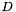Chombo + EB + MF  3.2
ConstantScalarFunction Class Reference

#include <ConstantScalarFunction.H>

Inheritance diagram for ConstantScalarFunction:[legend]

Public Member Functions

ConstantScalarFunction (Real a_value)

~ConstantScalarFunction ()
Destructor. More...

Real operator() (const RealVect &a_x, Real a_t) const

Real derivative (const IntVect &a_order, const RealVect &a_x, Real a_t) constPublic Member Functions inherited from ScalarFunction
ScalarFunction (bool a_homogeneous, bool a_constant)

virtual ~ScalarFunction ()
Destructor. More...

virtual bool hasDerivative (const IntVect &a_order) const

Real operator() (const RealVect &a_x) const

Real derivative (const IntVect &a_order, const RealVect &a_x) const

bool isHomogeneous () const
Returns true if this function is homogeneous, false otherwise. More...

bool isConstant () const
Returns true if this function is constant, false otherwise. More...

Private Attributes

Real m_valueProtected Attributes inherited from ScalarFunction
bool m_isHomogeneous

bool m_isConstant

Detailed Description

This ScalarFunction subclass represents a function that returns a constant value everywhere in space and time.

Constructor & Destructor Documentation

 ConstantScalarFunction::ConstantScalarFunction ( Real a_value )
explicit

Creates a constant scalar function returning the given value.

Parameters
 a_value The value returned by the function.
 ConstantScalarFunction::~ConstantScalarFunction ( )

Destructor.

Member Function Documentation

 Real ConstantScalarFunction::operator() ( const RealVect & a_x, Real a_t ) const
virtual

Override this method to evaluate this function at the given point in space and time.

Parameters
 a_x A point in-dimensional space. a_t The time at which the function is to be evaluated.

Implements ScalarFunction.

 Real ConstantScalarFunction::derivative ( const IntVect & a_order, const RealVect & a_x, Real a_t ) const
virtual

Override this method to evaluate the given partial derivative of the function at the given point in space and time.

Parameters
 a_order A multi-index identifying the order(s) of the partial derivative of the function to be evaluated. a_x A point in-dimensional space. a_t The time at which the derivative is to be evaluated.

Reimplemented from ScalarFunction.

Member Data Documentation

 Real ConstantScalarFunction::m_value
private

The documentation for this class was generated from the following file: